Ex 3.1

Chapter 3 Class 10 Pair of Linear Equations in Two Variables
Serial order wise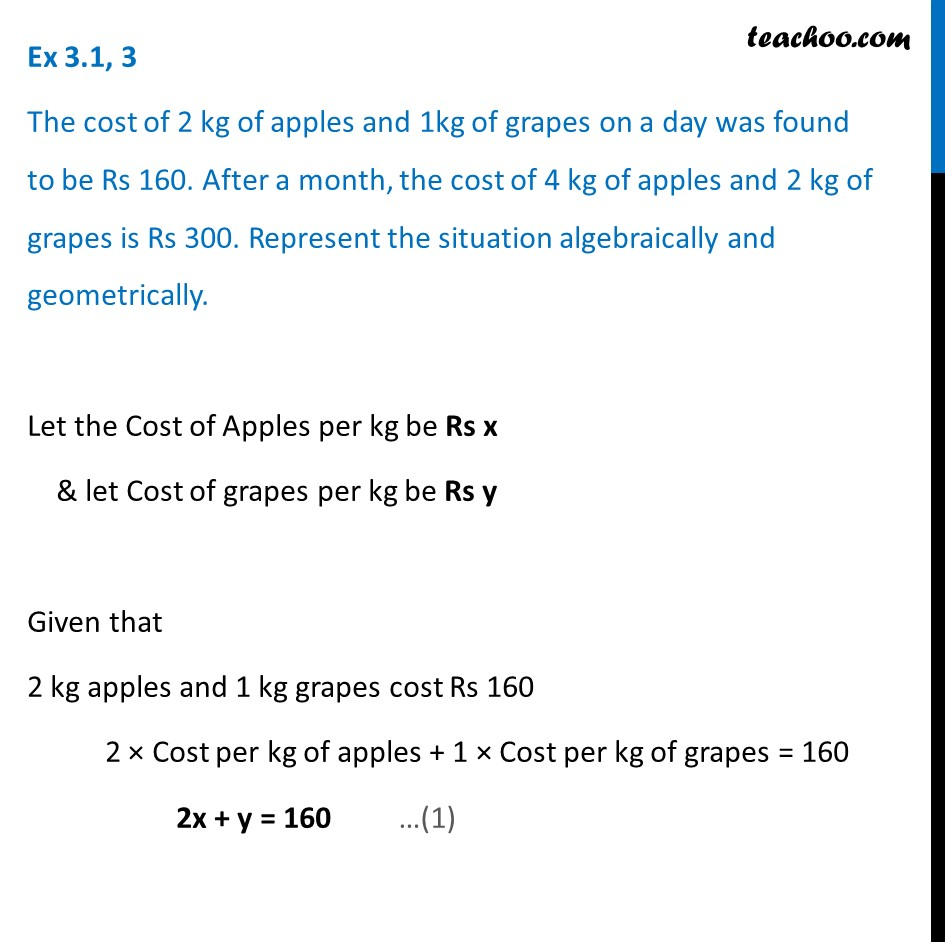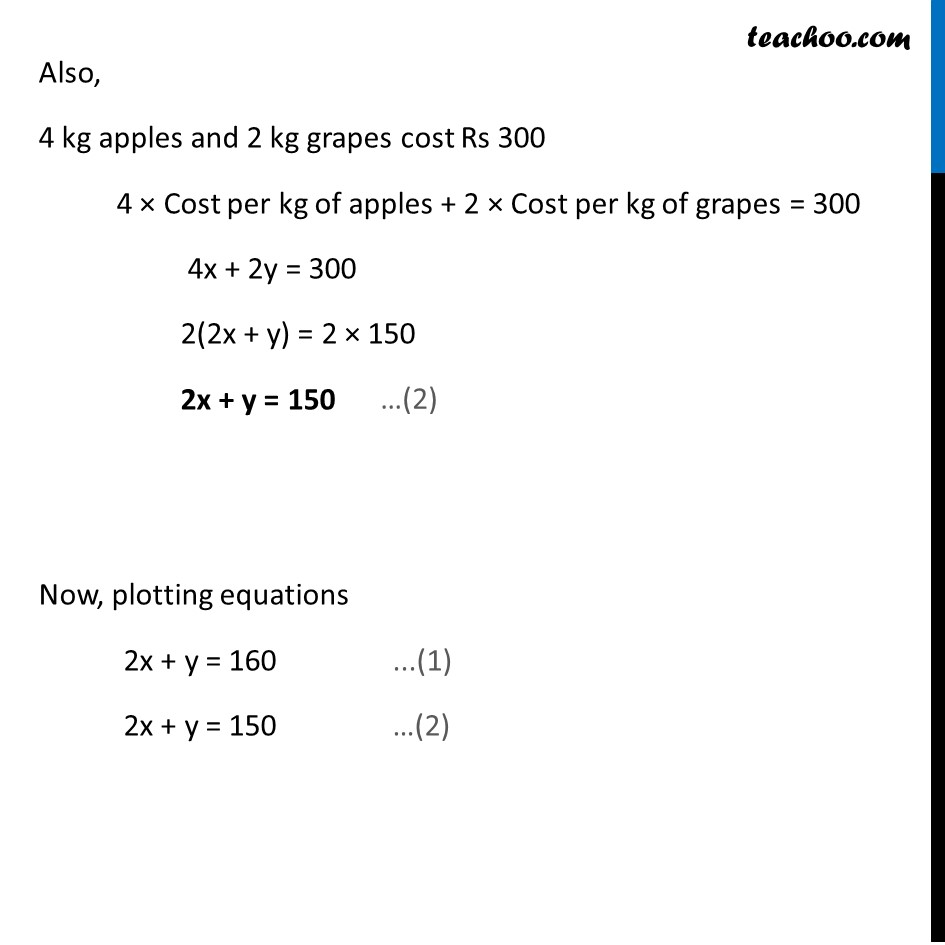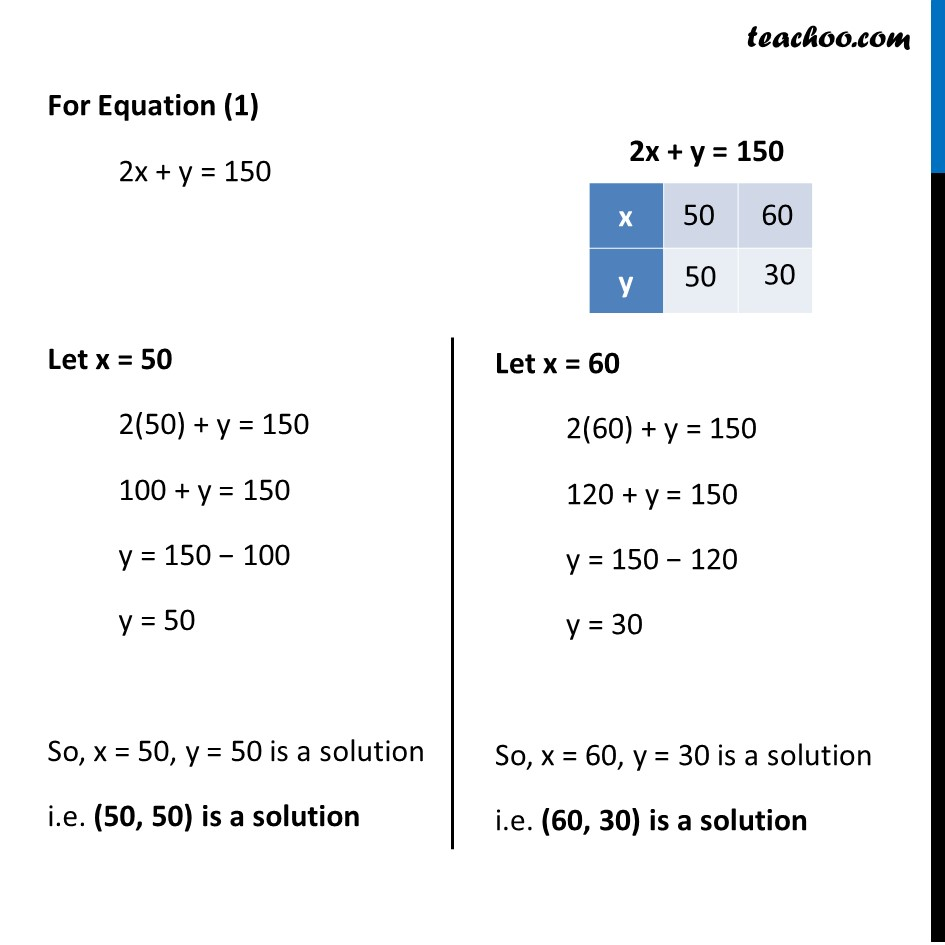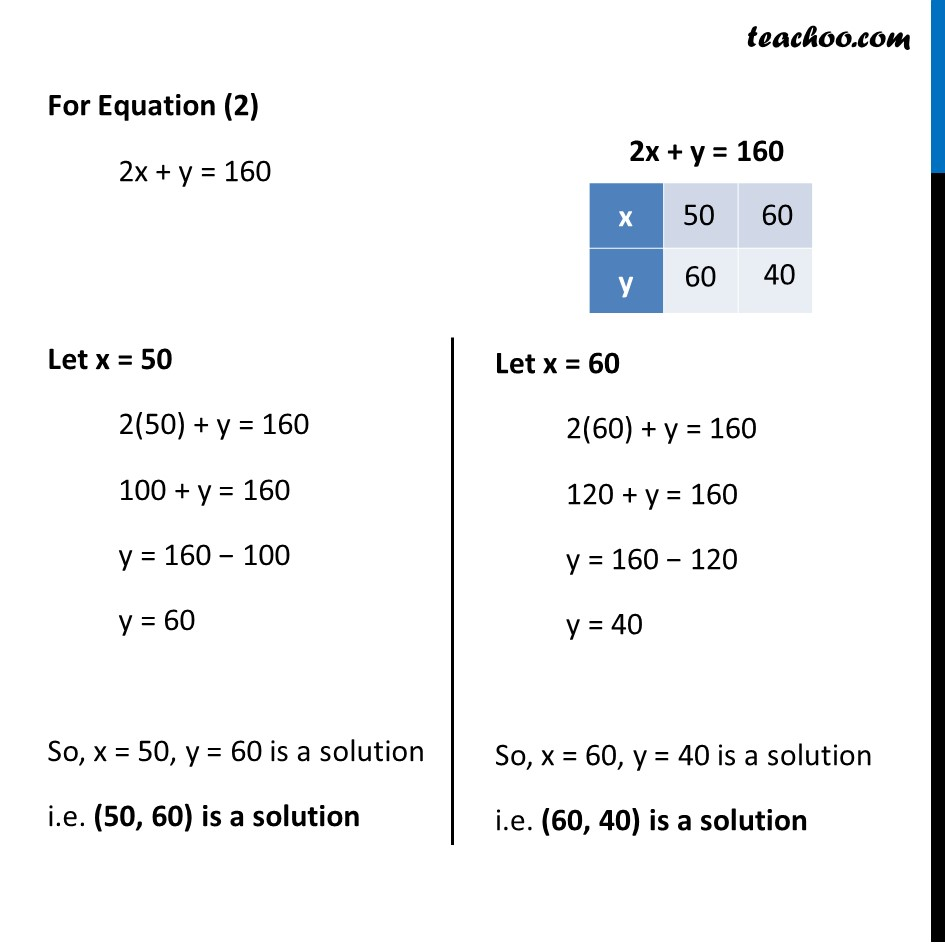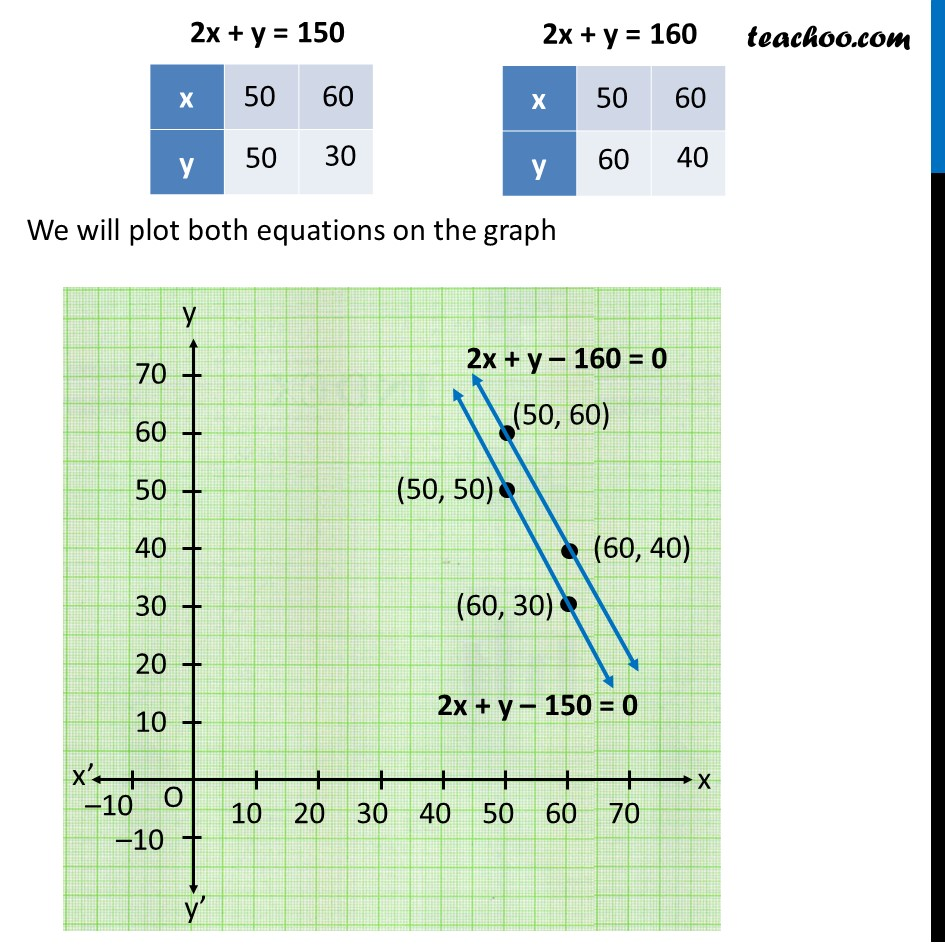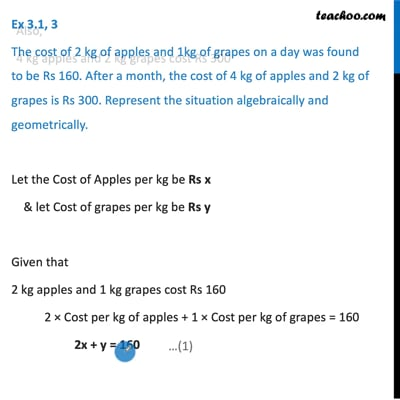This video is only available for Teachoo black users

Solve all your doubts with Teachoo Black (new monthly pack available now!)

### Transcript

Ex 3.1, 3 The cost of 2 kg of apples and 1kg of grapes on a day was found to be Rs 160. After a month, the cost of 4 kg of apples and 2 kg of grapes is Rs 300. Represent the situation algebraically and geometrically. Let the Cost of Apples per kg be Rs x & let Cost of grapes per kg be Rs y Given that 2 kg apples and 1 kg grapes cost Rs 160 2 × Cost per kg of apples + 1 × Cost per kg of grapes = 160 2x + y = 160 Also, 4 kg apples and 2 kg grapes cost Rs 300 4 × Cost per kg of apples + 2 × Cost per kg of grapes = 300 4x + 2y = 300 2(2x + y) = 2 × 150 2x + y = 150 Now, plotting equations 2x + y = 160 ...(1) 2x + y = 150 …(2) For Equation (1) 2x + y = 150 Let x = 50 2(50) + y = 150 100 + y = 150 y = 150 − 100 y = 50 So, x = 50, y = 50 is a solution i.e. (50, 50) is a solution Let x = 60 2(60) + y = 150 120 + y = 150 y = 150 − 120 y = 30 So, x = 60, y = 30 is a solution i.e. (60, 30) is a solution For Equation (2) 2x + y = 160 Let x = 50 2(50) + y = 160 100 + y = 160 y = 160 − 100 y = 60 So, x = 50, y = 60 is a solution i.e. (50, 60) is a solution Let x = 60 2(60) + y = 160 120 + y = 160 y = 160 − 120 y = 40 So, x = 60, y = 40 is a solution i.e. (60, 40) is a solution We will plot both equations on the graph